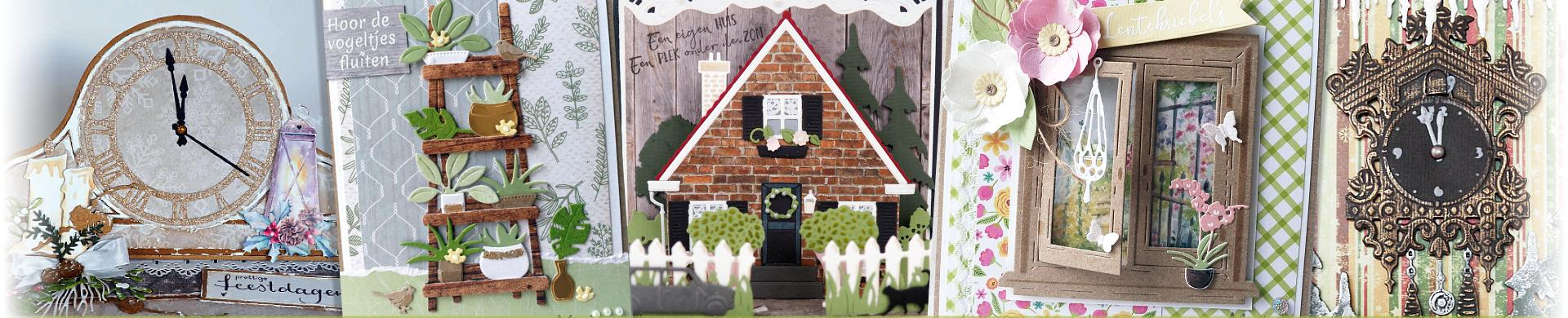# Cutting Dies - House & HomeHouse & Home themed thin metal cutting dies for card making and papercrafts.
• Brand
1. \$5.79
2. \$10.62
3. \$5.79
4. \$6.76
5. \$6.76
6. \$6.76
7. \$5.79
8. \$6.76
9. \$5.79
10. \$15.46
11. \$8.40
12. \$12.56
13. \$3.38
14. \$6.76
15. \$10.14
16. \$4.15
17. \$4.15
18. \$5.12
19. \$6.76
20. \$4.82
21. \$6.76
22. \$11.59
23. \$6.18
24. \$8.69
25. \$7.72
26. \$5.21
27. \$11.21
28. \$17.39
29. \$8.69
30. \$7.53
31. \$8.69
32. \$8.02
33. \$10.82
34. \$5.12
35. \$9.18
36. \$8.69
37. \$5.79
38. \$7.72
39. \$7.72
40. \$8.69
41. \$15.94
42. \$7.72
43. \$11.59
44. \$7.72
45. \$14.01
46. \$10.62
47. \$8.69
48. \$6.76
49. \$3.86
50. \$4.82
51. \$7.14
52. \$7.72
53. \$12.56
54. \$11.59
55. \$9.66
56. \$9.18
57. \$6.28
58. \$6.47
59. \$8.69
60. \$3.86
61. \$7.72
62. \$6.28
63. \$10.62
64. \$9.66
65. \$14.01
66. \$19.32
67. \$19.32
68. \$6.76
69. \$8.69
70. \$3.86
71. \$3.57
72. \$6.76